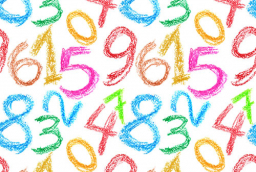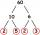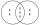# A number 5

A number is divisible by 24, 25, and 120 if it is increased by 20. Find the number.

x =  580

### Step-by-step explanation:Did you find an error or inaccuracy? Feel free to write us. Thank you!Tips to related online calculators
Do you want to calculate least common multiple two or more numbers?

## Related math problems and questions:

• Lcm of three numbersWhat is the Lcm of 120 15 and 5
• Twenty-fiveHow many are three-digit natural numbers divisible by 25?
• MO C–I–1 2018An unknown number is divisible by just four numbers from the set {6, 15, 20, 21, 70}. Determine which ones.
• Lcm simpleFind least common multiple of this two numbers: 140 175.
• ValueDetermine the value of the following exspressions: a) (23-25)·(4-5) b) (97-123):(18+8)
• Lcm to eliminate yIdentify the least common multiple that would eliminate the y-variable. 6x - 5y = -4 4x + 2y = 28
• Prime divisorsFind 2/3 of the sum's ratio and the product of all prime divisors of the number 120.
• LCMWhat is the least common multiple of 5, 50, 14?
• Bus linesAt 5.00 in the morning, four buses left the terminal at the same time. The first bus has an interval of 15 minutes, the second 20 minutes, the third 25 minutes, and the fourth 45 minutes. How many minutes will all four buses leave the terminal at the same
• Counting numberWhat is the smallest counting number divisible by 2,5,7,8 and 15?
• Lcm 2Create the smallest possible number that is divisible by numbers 5,8,9,4,3
• Find unknown numberWhat is the number between 50 and 55 that is divisible by 2,3,6,9?
• Equivalent expressionPablo rewrites the expression 8y-24 as 8(y-3). Hana rewrites 8y-24 as 2(4y-12). Are Pablo’s and Hana’s expressions each equivalent to 8y-24? Explain your reasoning.
• Reminder and quotientThere are given numbers A = 135, B = 315. Find the smallest natural number R greater than 1 so that the proportions R:A, R:B are with the remainder 1.
• Unknown number 24f we add 20, we get 50% of its triple. What is this unknown number?
• Apples and pearsMom divided 24 apples and 15 pears to children. Each child received the same number of apples and pears - same number as his siblings. How many apples (j=?) and pears (h=?) received each child?
• QuotientFind quotient before the bracket - the largest divisor 51 a + 34 b + 68 121y-99z-33